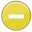#wavelength 中文解釋 wordnet sense Collocation Usage Collins Definition
Noun
/ˈwāvˌleNG(k)TH/,Font size:wavelengths, plural;
1. The distance between successive crests of a wave, esp. points in a sound wave or electromagnetic wave

2. This distance as a distinctive feature of radio waves from a transmitter

3. A person's ideas and way of thinking, esp. as it affects their ability to communicate with others
• - when we met we hit it off immediately—we're on the same wavelength

1. the distance (measured in the direction of propagation) between two points in the same phase in consecutive cycles of a wave
2. a shared orientation leading to mutual understanding; "they are on the same wavelength"
3. In physics, the wavelength of a sinusoidal wave is the spatial period of the wave – the distance over which the wave's shape repeats.
4. Wavelength is a forty-five minute film that made the reputation of Canadian experimental filmmaker and artist Michael Snow. Considered a landmark of avant-garde cinema, it was filmed over one week in December 1966 and edited in 1967 and is an example of what film theorist P. ...
5. Wavelength, the tenth album by Northern Irish singer-songwriter Van Morrison was released in the spring of 1978. The album has a different musical sound than his previous albums, leaning towards a pop sound with prominent electric guitars and synthesizers. ...
6. Multiple publications exist under the name Wavelength Magazine. 'Wavelength' is a worldwide surfing magazine published by Cornwall & Devon Media, based in Truro, Cornwall. ...
7. Wavelength (1984) is a soundtrack album by the German band Tangerine Dream for the film Wavelength starring Robert Carradine, Cherie Currie and Keenan Wynn.
8. The length of a single cycle of a wave, as measured by the distance between one peak or trough of a wave and the next; it is often designated in physics as λ, and corresponds to the velocity of the wave divided by its frequency
9. Distance, from peak to peak, of any waveform. For electromagnetic radiation in the infrared region, typically measured in microns and symbolized by l.
10. The distance between points of corresponding phase of two consecutive cycles of a wave.
11. The distance between two neighboring crests of a traveling wave. The wavelength of light is between 400 and 700 nanometers.
12. Distance between two points of corresponding phase and is equal to waveform velocity divided by frequency.
13. The distance between consecutive crests of a wave. This serves as a unit of measure of electromagnetic radiation.
14. The distance that an electromagnetic wave travels in one complete cycle.
15. Electromagnetic energy is transmitted in the form of a sinusoidal wave. The wavelength is the physical distance covered by one cycle of this wave; it is inversely proportional to frequency.
16. The wavelength is the distance between repeating units of a wave pattern. It is commonly designated by the Greek letter lambda (λ).
17. The distance between the top of one wave and the top of the next wave. The argon fluoride excimer wavelength is 193 nm. This wavelength is in the far ultraviolet end of the electromagnetic spectrum.
18. The distance between two crests (or valleys) of sound wave pressure. Easy to see graphically (5.2 kb). Usually denoted by the symbol λ. Varies from 56 feet for a 20 Hz tone to 0.7 inches for a 20 kHz tone. In general the wavelength in inches is equal to 13,543 divided by the frequency in Hz.
19. All electromagnetic radiation can be thought of a sinusoidal wave traveling through space, which each of the different bands having a unique wavelength. This is the length between two consecutive peaks or troughs.
20. The length of one wave cycle of a light wave. It is most commonly expressed in nanometers (nm) for the visible and UV, and “microns” (10nm/mm) in the IR spectral regions. The frequency (and also bandwidth) is inversely proportional to the wavelength.
21. The distance between adjacent crests or troughs of a light wave, which determines its color. The optics in NIF's main laser produce infrared laser light with a wavelength of 1,053 nanometers (billionths of a meter), also known as one-omega (1ω). ...
22. Commonly referred to as a Wave. Wavelength (i.e. color of light) is the distance between identical points in the adjacent cycles of a waveform signal propagated in space or along a wire.
23. Usually measured from peak to peak or trough to trough
24. The physical length of a complete period of sinusoidal radio wave.  Usually measure in meters or centimetres for long and short wavelengths respectively.  Used colloquially as the "position" of a station on a tuning scale. ...
25. The longitudinal distance from one peak to another on a wave form. For light, this corresponds to the speed of lightdivided by the radiation frequency. The visible spectrum begins in the infrared at 780 nanometers and continues into the ultraviolet at 350 nanometers.